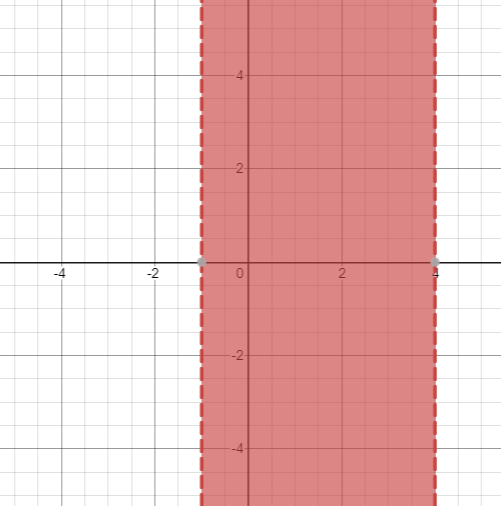# The solution of given nonlinear inequality equation and plot a graph for it.### Precalculus: Mathematics for Calcu...

6th Edition
Stewart + 5 others
Publisher: Cengage Learning
ISBN: 9780840068071### Precalculus: Mathematics for Calcu...

6th Edition
Stewart + 5 others
Publisher: Cengage Learning
ISBN: 9780840068071

#### Solutions

Chapter 1.7, Problem 45E
To determine

## The solution of given nonlinear inequality equation and plot a graph for it.

Expert Solution

1<x<4

### Explanation of Solution

Given:

Non-linear inequality equation

3x23x<2x2+4

Calculation,

Non-linear inequality equation,

3x23x<2x2+43x22x23x4>0x23x4>0x2(41)x4>0x24x+x4>0x(x4)+1(x4)>0(x+1)(x4)>0Now,(x+1)>0x>1and,(x4)>0x<4so,1<x<4

Now, graph of 3x23x<2x2+4Conclusion:

Hence, the values of x lies between the -1 and 4.

### Have a homework question?

Subscribe to bartleby learn! Ask subject matter experts 30 homework questions each month. Plus, you’ll have access to millions of step-by-step textbook answers!